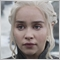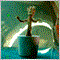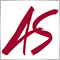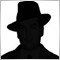• 概述
• 评论 (4)
• 评论 (90)
• 新特性

# Monday

The Monday EA trades on Mondays depending on price movement on Fridays. Its aim is to take 10-20 points of profit and leave the market.

This strategy works from March 2014.

• Currency pairs are EUR/USD and GBP/USD. For GBP / USD trading on the CCI indicator is recommended not to use
• Time frame is daily D1

### Settings:

• Sig_Open1 = true; - if true, the EA trades on Mondays
• StartTime = "00:00"; time to start trading (applies only to "Sig_Open1")
• Sig_Open2 = true; - enable only on EUR/USD (works only on EUR/USD).

If the CCI indicator crosses the CCIup value from the bottom upwards on the current daily candle, a buy order with predefined stop loss and take profit is opened. If the CCI indicator crosses the CCIdowm value downwards on the current daily candle, a sell order with predefined stop loss and take profit is opened. When the order is opened, the EA remembers that there is the opened order on the current candle and it will not open other orders there.

• CCI_TF=PERIOD_D1; - time frame of the CCI indicator, set irrespective of TF of the chart where the EA is attached to
• CCIPeriod=14; - CCI period
• CCIup = 0; - CCI upper bound line
• CCIdown = 0; - CCI lower bound line
• SL = 150; - stop loss
• TP = 10; - take profit

### Money Management:

• Lots = 0.01; fixed lot
• Use_Risk = true; - enable proportional lot calculation by parameter "Risk"
• Risk=20; -  lot is calculated according to percentage ratio of the deposit. Example: each 10 percents represent 0.01 lot per each 100 units of the deposit base currency. The EA also considers lot of the last closed order with the EA's Magic number: if calculated lot is smaller than the last closed order, the next order lot will be equal to the last closed order lot. If calculated lot is bigger than the last closed order, the next order lot will be equal to calculated lot. This is necessary in case that a user is going to trade aggressively.

Trailing stop

• Trailing_Use = false; use trailing stop
• Profit_Level_Trailing=100;  if the order has profit of the stated number of points.
• TrailingStop=50;  than the EA will trail stop loss following the price at a specified distance in points
• TrailingStep=50; modification step
```Breakeven
```
• Breakeven_Use=false; Use breakeven
• Profit_Level = 30; - if the order has profit of the stated number of points.
• SL_Plus = 1; - than stop loss is placed in profit on the stated number of points, i.e. we fix the profit by stop loss and allow the order to grow further
• Slip = 3; - slippage
• Magic = 100; Magic number of orders for trading on Monday
• Magic = 101; Magic number of orders for trading on the CCI indicator2048
2019.08.05 14:04

Small gain for great stop loss.189
2019.07.28 14:52

用户没有留下任何评级信息1027
2019.05.13 20:50